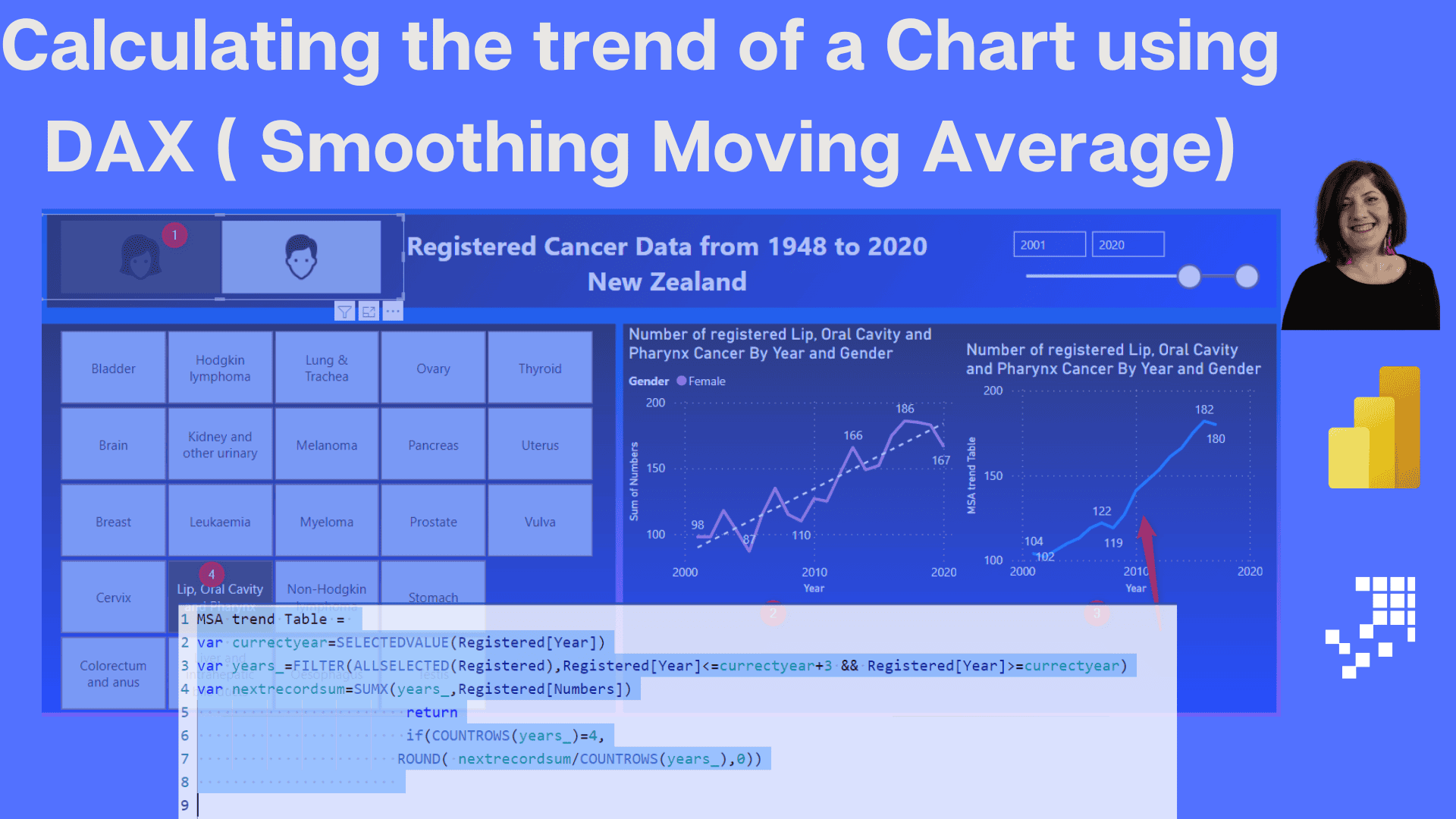# Calculating the trend of a Chart using DAX ( Smoothing Moving Average)

Calculating the trend of a line chart can be possible using the Analytics tab under the format section of the chart. What if we need to store this calculation and reuse it or show it as a separate chart? Actual Chart as below The trend of the chart The first question is about calculating the Read more about Calculating the trend of a Chart using DAX ( Smoothing Moving Average)[…]